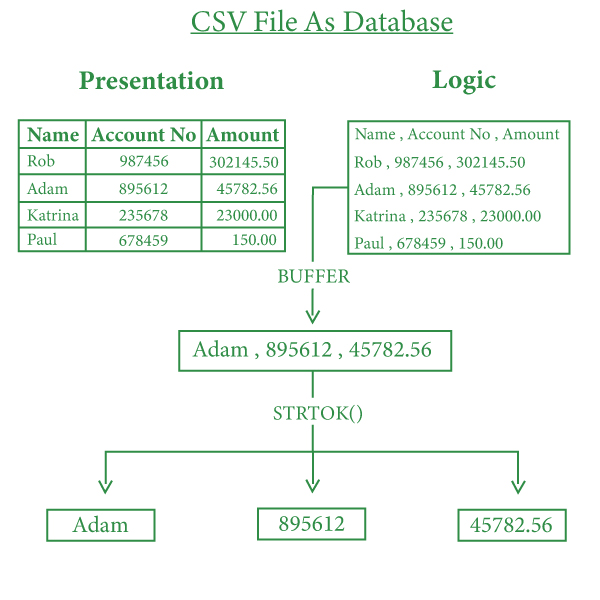# Relational Database from CSV Files in C

• Difficulty Level : Medium
• Last Updated : 24 Feb, 2022

In C programming, using arrays and string for data storage at run time which is volatile and gets memory in RAM. But to store data permanently in a hard disk which can be manipulated furthermore. So, the idea is to use a CSV file for data storage and manipulation. Not only CSV but other files like data, txt, and bin can also be used for data manipulation. But CSV file as the name suggests (Comma Separated Values) stores data in a table format which saves a lot of time in making perfect structure.

In Relational Databases data gets stored in a table format so by using CSV File, the database can be created.

Below is an example of a CSV File:For concepts of File Handling, refer to the Basic File Handling in C article.

Create a buffer of character array (can be referred to as string) which takes all the data present in the file and by using File Pointer and fgets() data can be extracted. Use two variables row and column which will maintain the unique identification of every entry.

As the string contains comma ‘, ‘ for separating values, so the idea is to use strtok() function for splitting values. This function splits a string using a delimiter here we are using ‘, ‘.

### Data Extraction:

Data Extraction deals with Opening an existing CSV file and extracting and printing the whole data on the console.

Approach:

1. Open CSV File using File Pointer.
2. Extract the whole file data into a char buffer array.
3. Now initialize row and column variables with value 0.
4. Print data separated by a comma and increase the column variable.
5. When reached to the end of a row entry initialize column variable to 0 and increase row variable.
6. Repeat steps 4 and 5, till the pointer reaches the end of the file.
7. Close the file.

Below is the program for the same:

## C

 `// C program for the above approach``#include ``#include ``#include ` `// Driver Code``int` `main()``{``    ``// Substitute the full file path``    ``// for the string file_path``    ``FILE``* fp = ``fopen``(``"file_path"``, ``"r"``);` `    ``if` `(!fp)``        ``printf``(``"Can't open file\n"``);` `    ``else` `{``        ``// Here we have taken size of``        ``// array 1024 you can modify it``        ``char` `buffer;` `        ``int` `row = 0;``        ``int` `column = 0;` `        ``while` `(``fgets``(buffer,``                     ``1024, fp)) {``            ``column = 0;``            ``row++;` `            ``// To avoid printing of column``            ``// names in file can be changed``            ``// according to need``            ``if` `(row == 1)``                ``continue``;` `            ``// Splitting the data``            ``char``* value = ``strtok``(buffer, ``", "``);` `            ``while` `(value) {``                ``// Column 1``                ``if` `(column == 0) {``                    ``printf``(``"Name :"``);``                ``}` `                ``// Column 2``                ``if` `(column == 1) {``                    ``printf``(``"\tAccount No. :"``);``                ``}` `                ``// Column 3``                ``if` `(column == 2) {``                    ``printf``(``"\tAmount :"``);``                ``}` `                ``printf``(``"%s"``, value);``                ``value = ``strtok``(NULL, ``", "``);``                ``column++;``            ``}` `            ``printf``(``"\n"``);``        ``}` `        ``// Close the file``        ``fclose``(fp);``    ``}``    ``return` `0;``}`

Data Addition deals with opening an existing CSV file, taking user inputs for the data to be added to the file, and then adding this data to the CSV file.

Approach:

1. Open CSV File using File Pointer in append mode which will place a pointer to the end of the file.
2. Take Input from the user in temporary variables.
3. Use fprintf() and separate variables according to their order and comma.
4. Close the file.

Example:

## C

 `// C program for the above approach``#include ``#include ``#include ` `// Driver Code``int` `main()``{``    ``// Substitute the file_path string``    ``// with full path of CSV file``    ``FILE``* fp = ``fopen``(``"file_path"``, ``"a+"``);` `    ``char` `name;``    ``int` `accountno, amount;` `    ``if` `(!fp) {``        ``// Error in file opening``        ``printf``(``"Can't open file\n"``);``        ``return` `0;``    ``}` `    ``// Asking user input for the``    ``// new record to be added``    ``printf``(``"\nEnter Account Holder Name\n"``);``    ``scanf``(``"%s"``, &name);``    ``printf``(``"\nEnter Account Number\n"``);``    ``scanf``(``"%d"``, &accountno);``    ``printf``(``"\nEnter Available Amount\n"``);``    ``scanf``(``"%d"``, &amount);` `    ``// Saving data in file``    ``fprintf``(fp, ``"%s, %d, %d\n"``, name,``            ``accountno, amount);` `    ``printf``(``"\nNew Account added to record"``);` `    ``fclose``(fp);``    ``return` `0;``}`

Output:

### Advantages Of CSV File:

• Different from .txt and .dat file in terms of storing data in table format.
• Easy to organize data by direct user interaction or by the program.
• Widely adopted in financial industries to store and transmit data over the internet.
• Easily converted to other files and formats.
• It can be imported or exported to various platforms and interfaces.

My Personal Notes arrow_drop_up